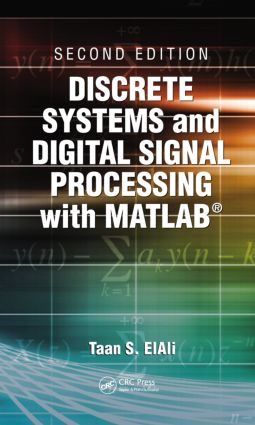Discrete Systems and Digital Signal Processing with MATLAB

2nd Edition

CRC Press

620 pages | 307 B/W Illus.

Hardback: 9781439828182
pub: 2011-12-05
\$130.00
x
eBook (VitalSource) : 9780429112928
pub: 2016-04-19
from \$65.00

FREE Standard Shipping!

Description

"Discrete linear systems and digital signal processing have been treated for years in separate publications. ElAli has skillfully combined these two subjects into a single and very useful volume. … Useful for electrical and computer engineering students and working professionals… a nice addition to the shelves of academic and public libraries.

"Summing Up: Highly Recommended."

— S.T. Karris, University of California, Berkeley in CHOICE

Typically, books on linear systems combine coverage of both discrete and continuous systems all in a single volume. The result is usually a daunting mountain of information that fails to sufficiently explain either subject. With this in mind, Discrete Systems and Digital Signal Processing with MATLAB®, Second Edition responds to the need in engineering for a text that provides complete, focused coverage of discrete linear systems and associated problem solution methods.

With its simplified presentation, this book follows a logical development that builds on basic mathematical principles to cover both discrete linear systems and signal processing. The author covers all traditional topics and includes numerous examples that are solved analytically and, when applicable, numerically using the latest version of MATLAB®. In addition to the classical coverage, the author includes complete and stand-alone chapters on IIR and FIR filter design, block diagrams, state-space, and sampling and transformations, as well as a unique chapter on FFT and its many applications. The book also introduces many examples using the MATLAB data acquisition toolbox in different chapters.

Ideal either as a textbook for the required course in the electrical and computer engineering curriculum or as an updated refresher for seasoned engineers, this resource offers a wealth of examples, exercises, problems, and author insights.

Signal Representation

Introduction

Why Do We Discretize Continuous Systems?

Periodic and Nonperiodic Discrete Signals

Unit Step Discrete Signal

Impulse Discrete Signal

Ramp Discrete Signal

Real Exponential Discrete Signal

Sinusoidal Discrete Signal

Exponentially Modulated Sinusoidal Signal

Complex Periodic Discrete Signal

Shifting Operation

Representing a Discrete Signal Using Impulses

Reflection Operation

Time Scaling

Amplitude Scaling

Even and Odd Discrete Signal

Does a Discrete Signal Have a Time Constant?

Basic Operations on Discrete Signals

Energy and Power Discrete Signals

Bounded and Unbounded Discrete Signals

Some Insights: Signals in the Real World

Discrete System

Definition of a System

Input and Output

Linear Discrete Systems

Time Invariance and Discrete Signals

Systems with Memory

Causal Systems

Inverse of a System

Stable System

Convolution

Difference Equations of Physical Systems

Homogeneous Difference Equation and Its Solution

Nonhomogeneous Difference Equations and Their Solutions

Stability of Linear Discrete Systems: The Characteristic Equation

Block Diagram Representation of Linear Discrete Systems

From the Block Diagram to the Difference Equation

From the Difference Equation to the Block Diagram: A Formal Procedure

Impulse Response

Correlation

Some Insights

Fourier Series and the Fourier Transform of Discrete Signals

Introduction

Review of Complex Numbers

Fourier Series of Discrete Periodic Signals

Discrete System with Periodic Inputs: The Steady-State Response

Frequency Response of Discrete Systems

Fourier Transform of Discrete Signals

Convergence Conditions

Properties of the Fourier Transform of Discrete Signals

Parseval’s Relation and Energy Calculations

Numerical Evaluation of the Fourier Transform of Discrete Signals

Some Insights: Why Is This Fourier Transform?

z-Transform and Discrete Systems

Introduction

Bilateral z-Transform

Unilateral z-Transform

Convergence Considerations

Inverse z-Transform

Properties of the z-Transform

Representation of Transfer Functions as Block Diagrams

x(n), h(n), y(n), and the z-Transform

Solving Difference Equation Using the z-Transform

Convergence Revisited

Final-Value Theorem

Initial-Value Theorem

Some Insights: Poles and Zeroes

State-Space and Discrete Systems

Introduction

Review on Matrix Algebra

General Representation of Systems in State Space

Solution of the State-Space Equations in the z-Domain

General Solution of the State Equation in Real Time

Properties of An and Its Evaluation

Transformations for State-Space Representations

Some Insights: Poles and Stability

Block Diagrams and Review of Discrete System Representations

Introduction

Basic Block Diagram Components

Block Diagrams as Interconnected Subsystems

Controllable Canonical Form Block Diagrams with Basic Blocks

Observable Canonical Form Block Diagrams with Basic Blocks

Diagonal Form Block Diagrams with Basic Blocks

Parallel Block Diagrams with Subsystems

Series Block Diagrams with Subsystems

Block Diagram Reduction Rules

Discrete Fourier Transform and Discrete Systems

Introduction

Discrete Fourier Transform and the Finite-Duration Discrete Signals

Properties of the DFT

Relation the DFT Has with the Fourier Transform of Discrete Signals, the z-Transform, and the Continuous Fourier Transform

Numerical Computation of the DFT

Fast Fourier Transform: A Faster Way of Computing the DFT

Applications of the DFT

Some Insights

Sampling and Transformations

Need for Converting a Continuous Signal to a Discrete Signal

From the Continuous Signal to Its Binary Code Representation

From the Binary Code to the Continuous Signal

Sampling Operation

How Do We Discretize the Derivative Operation?

Discretization of the State-Space Representation

Bilinear Transformation and the Relationship between the Laplace-Domain and the z-Domain Representations

Other Transformation Methods

Some Insights

Infinite Impulse Response Filter Design

Introduction

Design Process

IIR Filter Design Using MATLAB®

Some Insights

Finite Impulse Response Digital Filters

Introduction

FIR Filter Design

Design Based on the Fourier Series: The Windowing Method

From IIR to FIR Digital Filters: An Approximation

Frequency Sampling and FIR Filter Design

FIR Digital Design Using MATLAB®

Some Insights

Bibliography

Index

Dr. Taan ElAli

Columbia,, South Carolina,, USA